﻿ 水下滑翔机动力学建模及PID控制
 舰船科学技术2017, Vol. 39Issue (12): 64-69PDF

Dynamic modeling and PID control of underwater glider
WANG Xu-chao, QI Xiang-dong
School of Electronics and Information, Taiyuan University of Science and Technology, Taiyuan 030051, China
Abstract: In order to solve the problem of underwater glider control, the tail four-propeller is designed to control the pitching and steering of the glider. The kinematics and dynamics of the underwater glider are modeled and the PID controller is designed on the basis of it. The validity of the designed PID controller is verified by Matlab/simulink simulation and lake test. The simulation results show that the controller can effectively control the self-stabilization of the underwater glider under the designed PID parameters. Compared with the simulation results, it can be concluded that the design of the PID controller is reasonable and the attitude can be adjusted quickly and accurately.
Key words: underwater glider     dynamic model     PID control     Matlab simulation     lake test
0 引　言

21世纪以来，海洋资源的开发和利用问题越来越引起人们的关注，随着技术的不断成熟，水下滑翔机作为一种新型的海洋勘测工具逐渐发展起来。水下滑翔机中的姿态调节单元能调整机体的俯仰角和偏转角，是AUV的重要运动功能单元，其设计的优劣直接影响AUV运动性能的好坏。传统的方式通过调整内部压载重块的位置，在浮力调节单元外皮囊体积变化和水平机翼的配合下，实现航行器转向运动。由于这种方法是间接通过改变重心来改变姿态的，这就导致控制过程中的不精确和不稳定性，尤其在受到外界干扰时，这种不稳定性会被放大，从而导致任务失败。针对水下滑翔机动力学的非线性、强耦合、模型参数复杂，因此设计了多桨驱动无舵水下滑翔机。通过4个螺旋桨的互相协调配合，直接改变转速来改变运动姿态，在建立了运动模型基础上，结合PID算法，设计合理的PID控制器，用仿真来验证算法的稳定性和抗干扰能力，并通过试验来验证这种方法的有效性。

1 结构及其工作原理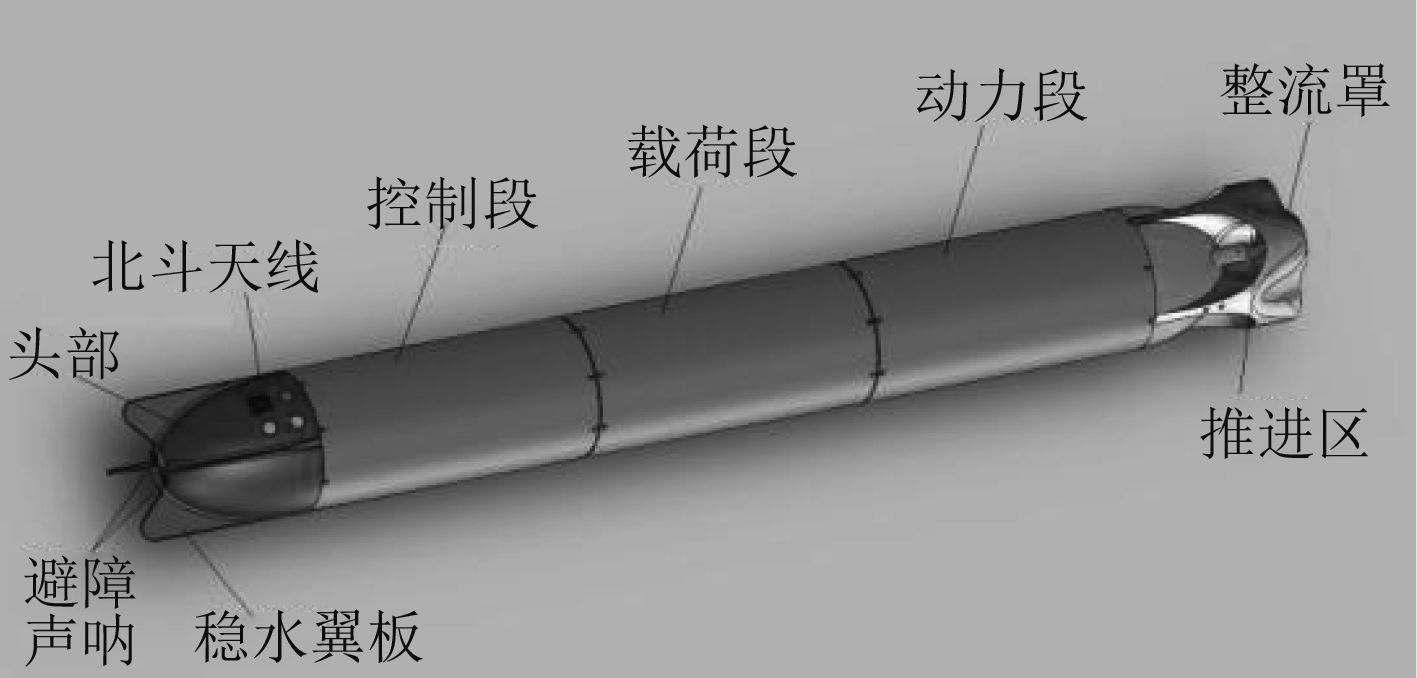图 1 滑翔机整体结构图 Fig. 1 Overall structure of the glider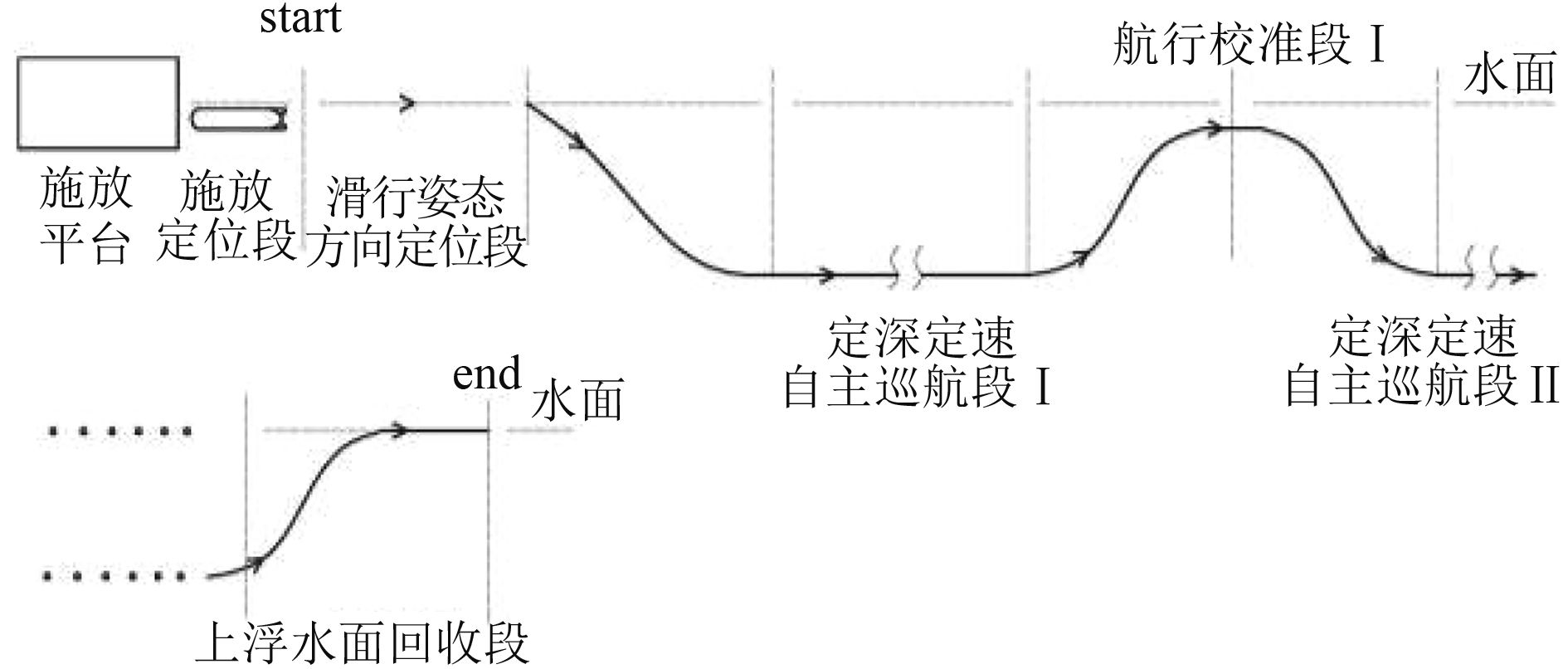图 2 滑翔机运动示意图 Fig. 2 Glider motion diagram
2 滑翔机动力学模型的建立

2.1 坐标系的选择和建立

2.1.1 地面坐标系

2.1.2 载体坐标系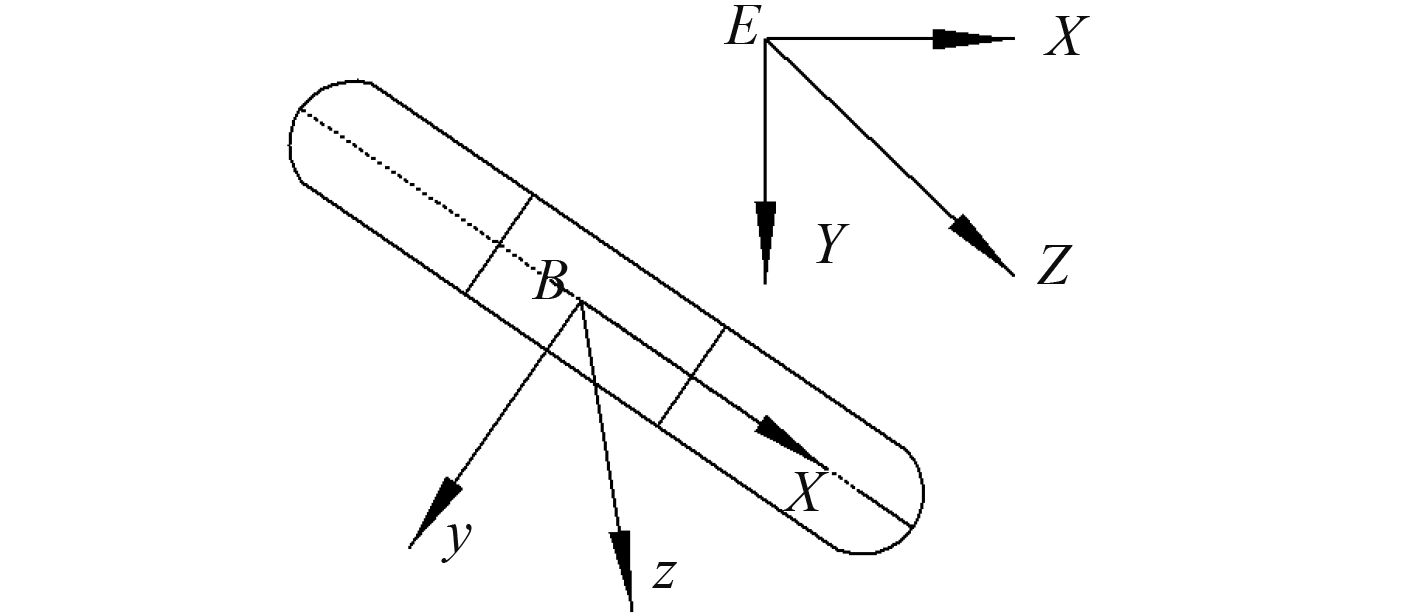图 3 滑翔机坐标系的建立 Fig. 3 The establishment of the glider coordinate system

2个坐标系之间的向量变化需要通过旋转矩阵来变换，其变换矩阵为：

 $\begin{split}R_B^E = \left[ {\begin{array}{*{20}{l}}{\cos \psi \cos \theta }&{\sin \theta }\\{\sin \psi \sin \phi - \cos \psi \sin \theta \cos \varphi }&{\cos \theta \cos \varphi }\\{\cos \psi \sin \theta \sin \phi + \sin \psi \cos \varphi }&{ - \cos \theta \sin \varphi }\end{array}} \right.\\\left. {\begin{array}{*{20}{c}}{ - \sin \psi \cos \theta }\\{\sin \theta \sin \psi \cos \varphi + \cos \psi \sin \varphi }\\{\cos \psi \cos \varphi - \sin \psi \sin \theta \sin \varphi }\end{array}} \right]\text{，}\end{split}$ (1)

2.2 四螺旋桨原理分析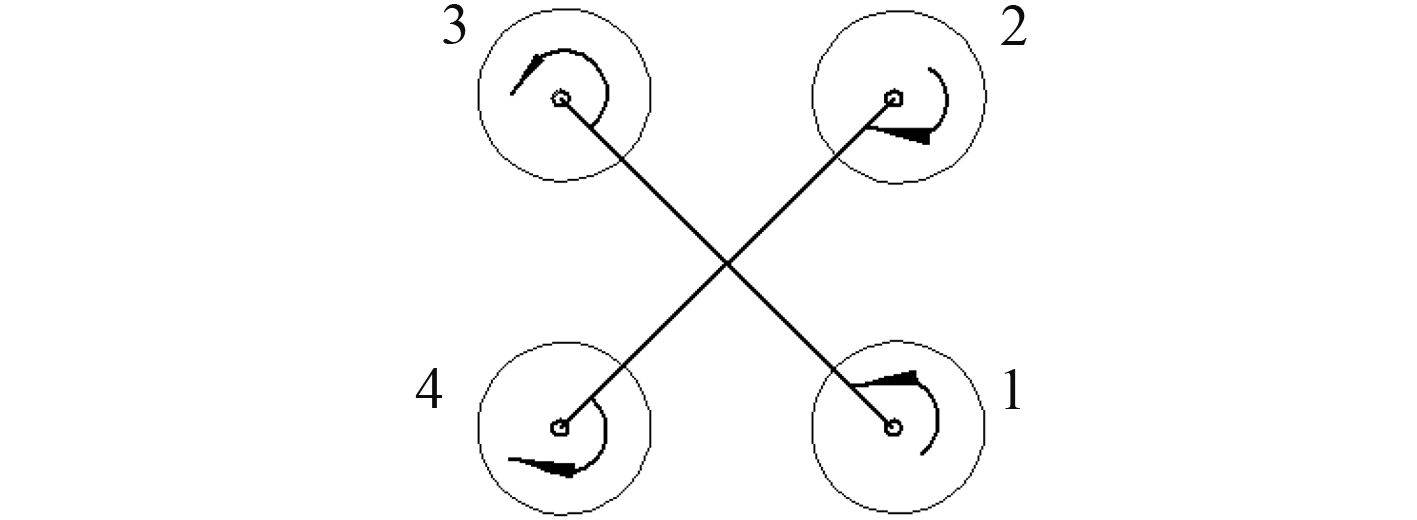图 4 螺旋桨旋转方向图 Fig. 4 Propeller rotation pattern
2.3 运动方程建立

2.3.1 平移运动方程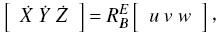(2)
 $\left\{ \begin{array}{l}\dot X = u\cos \theta \cos \psi + v\sin \psi \sin \phi - v\sin \theta \cos \psi \cos \phi + \\w\sin \psi \cos \phi + w\sin \theta \cos \psi \sin \phi \\\dot Y = u\sin \theta + v\cos \theta \cos \phi - w\cos \theta \sin \phi \\\dot Z = - u\cos \theta \sin \psi + v\cos \psi \sin \phi + v\sin \theta \sin \psi \cos \phi + \\w\cos \psi \cos \phi - w\sin \theta \sin \psi \sin \varphi \end{array} \right\}\text{。}$ (3)
2.3.2 旋转运动方程

 ${\varOmega} = \left[ {\begin{array}{*{20}{c}}{\sin \theta }&0&1\\{\cos \theta \cos \phi }&{\sin \phi }&0\\{ - \cos \theta \sin \phi }&{\cos \phi }&0\end{array}} \right]\left[ {\begin{array}{*{20}{c}}{\dot \psi }\\{\dot \theta }\\{\dot \phi }\end{array}} \right]\text{。}$ (4)
2.4 滑翔机受力分析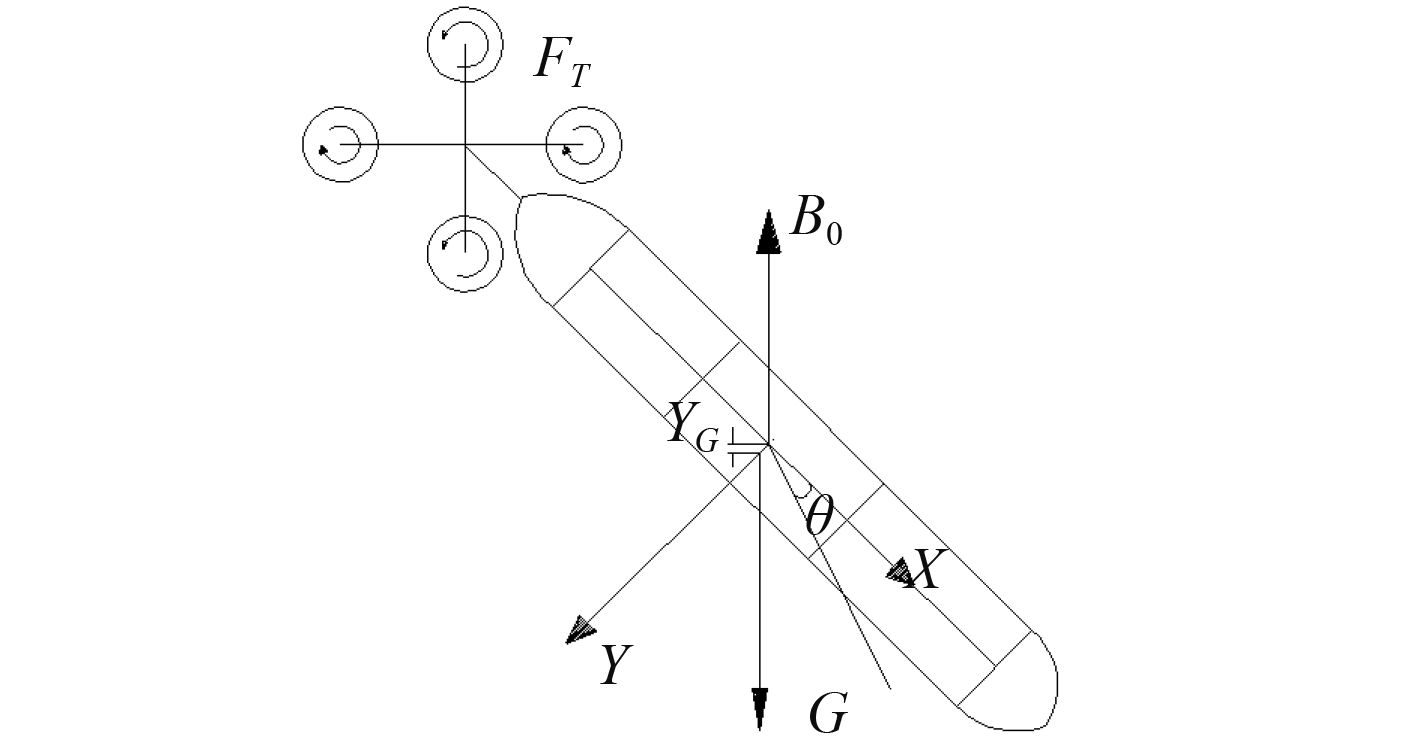图 5 滑翔机受力分析图 Fig. 5 Glider force analysis chart
2.4.1 重力浮力

 ${F_G} = R_B^E\left[ {\begin{array}{*{20}{c}}0\\G\\0\end{array}} \right] = G\left[ {\begin{array}{*{20}{c}}{\sin \theta }\\{\cos \theta \cos \phi }\\{ - \cos \theta \sin \phi }\end{array}} \right]\text{，}$ (5)
 ${F_B} = R_B^E\left[ {\begin{array}{*{20}{c}}0\\{ - {B_{}}}\\0\end{array}} \right] = {B_{}}\left[ {\begin{array}{*{20}{c}}{ - \sin \theta }\\{ - \cos \theta \cos \phi }\\{\cos \theta \sin \phi }\end{array}} \right]\text{，}$ (6)
 $\begin{split}T{}_G = {r_G} \times {F_G} = G\left[ {\begin{array}{*{20}{c}}0\\{{y_G}}\\0\end{array}} \right] \times \left[ {\begin{array}{*{20}{c}}{\sin \theta }\\{\cos \theta \cos \phi }\\{ - \cos \theta \sin \phi }\end{array}} \right]=\\ G{y_G}\left[ {\begin{array}{*{20}{c}}{ - \cos \theta \sin \phi }\\0\\{ - \sin \theta }\end{array}} \right]\text{。}\end{split}$ (7)
2.4.2 螺旋桨推力与力矩

 $\left\{ \begin{array}{l}\begin{array}{*{20}{c}}\!\!\!\!\!\!\!\!{{F_t} = \rho Z\int_{{r_h}}^{_{{r_t}}} {\Gamma (r)({w_t}r - 0.5{u_t})(1 - \varepsilon tg{\beta _i}){\rm d}r} }\text{，}\\[5pt]\end{array}\\\!\!\!\!{T_t} = \rho Z\int_{{r_h}}^{{r_t}} {\Gamma (r)(V + 0.5{u_a})(1 + \varepsilon ctg{\beta _i})r{\rm d}r} \text{。}\end{array} \right.$ (8)

 $\left\{ {\begin{array}{*{20}{l}}{{F_t} = (1 - t){K_T}\rho {n^2}d_\rho ^4}\text{，}\\{{T_t} = {K_Q}\rho {n^2}d_p^5}\text{。}\end{array}} \right.$ (9)

 $\left[ {\begin{array}{*{20}{c}}{{F_T}}\\{{T_T}}\end{array}} \right] = \left[ {\begin{array}{*{20}{c}}{\displaystyle\sum\limits_{i = 1}^2 {{F_i}} }\\{\displaystyle\sum\limits_{i = 1}^2 {{T_i}} }\end{array}} \right]\left[ {\begin{array}{*{20}{c}}{({F_1} + {F_2} + {F_3} + {F_4})\cos \alpha }\\{({F_1} - {F_2} - {F_3} + {F_4})\sin \alpha }\\0\\0\\0\\{c({F_1} - {F_2} + {F_3} - {F_4})\sin \alpha }\end{array}} \right]\text{。}$ (10)
2.4.3 流体动态力

 $\begin{array}{l}F = \displaystyle\sum\limits_i {{F_i}} = {F_G} + {F_B} + {F_v} + {F_T} + {F_I}\text{，}\\T = \displaystyle\sum\limits_j {{T_j}} = {T_G} + {T_B} + {T_T} + {T_V} + {T_I}\text{。}\end{array}$ (11)
2.5 滑翔机动力学方程表 1 变量定义 Tab.1 Variable definitions

 $\begin{array}{l}\dot P = P \times \Omega + F\text{，}\\\dot L = l \times \Omega + R_B^E\displaystyle\sum\limits_{i = 1} {({d_i} \times f{}_i} ) + T\text{。}\end{array}$ (12)

 ${L_B} = {{J}_C}\varOmega = {I_{Cx}}pi + {I_{Cy}}qj + {I_{Cx}}rk\text{，}$ (13)

 $\Bigg\{ {} \begin{array}{*{20}{c}}\!\!{m(\dot V + \dot \varOmega \times {r_G} + \varOmega \times V + ({r_G} \times \varOmega ) \times \varOmega ) = F}\text{，}\\\!\!\!\!\!\!\!\!\!\!\!\!\!\!\!\!\!\!\!\!{{J_C}\dot \varOmega + {J_C}\varOmega \times \varOmega = R_B^E\displaystyle\sum\limits_{i = 1} {({d_i} \times {f_i}) + T} }\text{。}\end{array}$ (14)

 $\begin{array}{c}\!\!\!\!\!\!\!\! \left\{ {\begin{array}{*{20}{l}}{\dot X = u\cos \theta \cos \psi \!\!+\!\! v\sin \psi \sin \phi \!-\! v\sin \theta \cos \psi \cos \phi }\text{，}\!\!\\{\dot Y = u\sin \theta + v\cos \theta \cos \phi - w\cos \theta \sin \phi }\text{，}\\{\dot Z = - u\cos \theta \sin \psi \!\!+\!\! v\cos \psi \sin \phi \!\!+\!\! v\sin \theta \sin \psi \cos \phi }\text{，}\!\!\!\\{\dot \psi = q\cos \phi /\cos \theta - r\sin \phi /\cos \theta }\text{，}\\{\dot \theta = q\sin \phi + r\cos \phi }\text{，}\\{\dot \phi = p - q\cos \phi \tan \theta + r\sin \phi \tan \theta +}\end{array}} \right.\\\begin{array}{*{20}{c}}{ w\sin \psi \cos \phi + w\sin \theta \cos \psi \sin \phi }+\\{ w\sin \psi \cos \phi + w\sin \theta \cos \psi \sin \phi }\text{。}\end{array}\end{array}\!\!\!\!\!\!\!\!$ (15)
 $\begin{array}{l}\left\{ {\begin{array}{*{20}{l}}{m\dot u - m{y_G} - mv\dot r + mwq + m{y_G}pq = }\\{m\dot v + \dot r - mwp + mur - m{y_G}({r^2} + {p^2}) = }\\{m\dot \omega - \dot q + m{y_G}\dot p - muq + mvp + m{y_G}rq = }\\{{J_{Bx}}\dot p \!+\! m{y_G}(vp \!+\! \dot w - uq) \!+\! ({J_{Bs}} \!-\! {J_{By}})qr \!+\! G{y_G}\cos \theta \sin \phi }=\\{ {J_{By}}\dot q + \dot w + ({J_{Bx}} - {J_{BG}})rp = (mg - b)\cos \theta \sin \phi }\\{{J_s}\dot r + \dot v + m{y_G}(wq + \dot u - vr) + ({J_{By}} - {J_{Bx}})pq - }\end{array}} \right.\\\begin{array}{*{20}{l}}{ {B_b}\sin \theta + (G - {B_0})\sin \theta + (1 - t){K_T}\rho {n^2}{d_p}^4}-\\{ {B_b}\cos \theta \cos \phi + (G - {B_0})\cos \theta \cos \phi }\\{{B_b}\cos \theta \sin \phi - (G - {B_0})\cos \theta \cos \phi }\\{{K_Q}\rho {n^2}{d_p}^5 + mg[(1-\cos \varphi )\cos \theta \sin \phi-\sin \varphi \cos \theta \cos \phi]}+\\{ mg\sin \varphi \sin \theta }\\{mg\cos \theta \cos \phi + G{y_G}\sin \theta }\text{。} \quad\quad\quad\quad\quad\quad\quad\quad\quad\quad\, \end{array}\end{array}$ (16)

3 PID控制器设计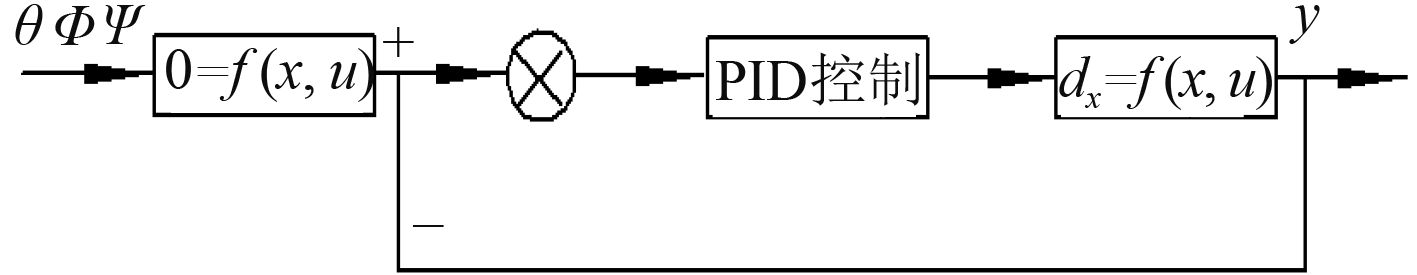图 6 控制系统框图 Fig. 6 Block diagram of the control system

 $\begin{array}{l}x = {(u, v, w, p, q, r, \theta, \phi, \varphi )^{\rm T}}\text{，}\\u = ({u_1}, {u_2}, {u_3}, {u_4})\text{。}\end{array}$ (17)

 $\Delta \dot x = A\Delta x + B\Delta u\text{，}$ (18)

 $u(t) = {K_P}\left\{ {e(t) + 1/{T_i}\int_0^t {e(t){\rm d}(t) + {T_{d}}\frac{{{\rm d}[e(t)]}}{{{{\rm d}_t}}}} } \right\}\text{，}$ (19)

 ${n_r} = {K_{pr}}e + {K_{ir}}\int_{{t_0}}^t {e(\tau ){\rm d}\tau + {K_{{ d}r}}\dot e} \text{。}$ (20)

4 Matlab/Simulink仿真

4.1 下潜与上浮过程仿真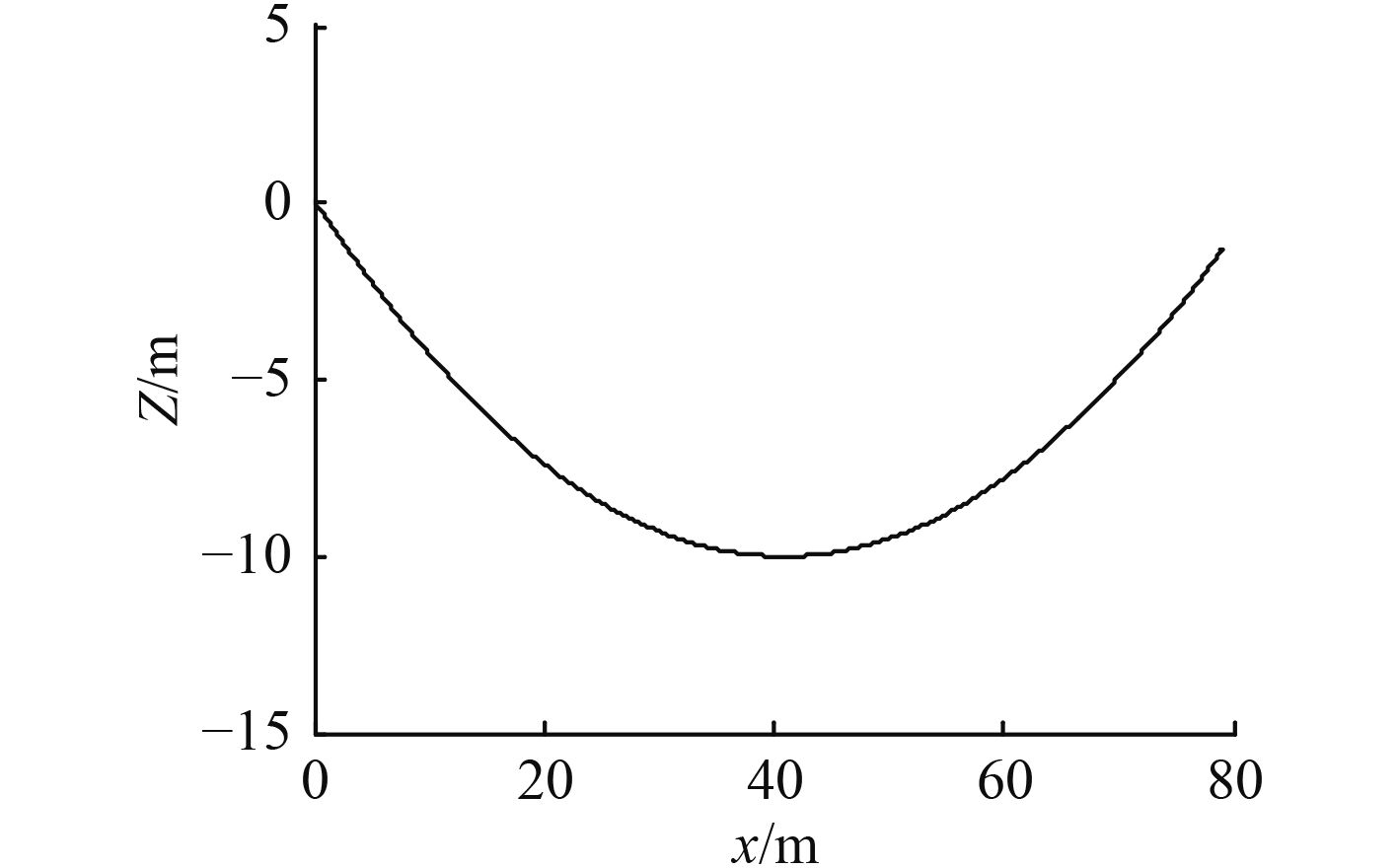图 7 航行轨迹图 Fig. 7 Sailing chart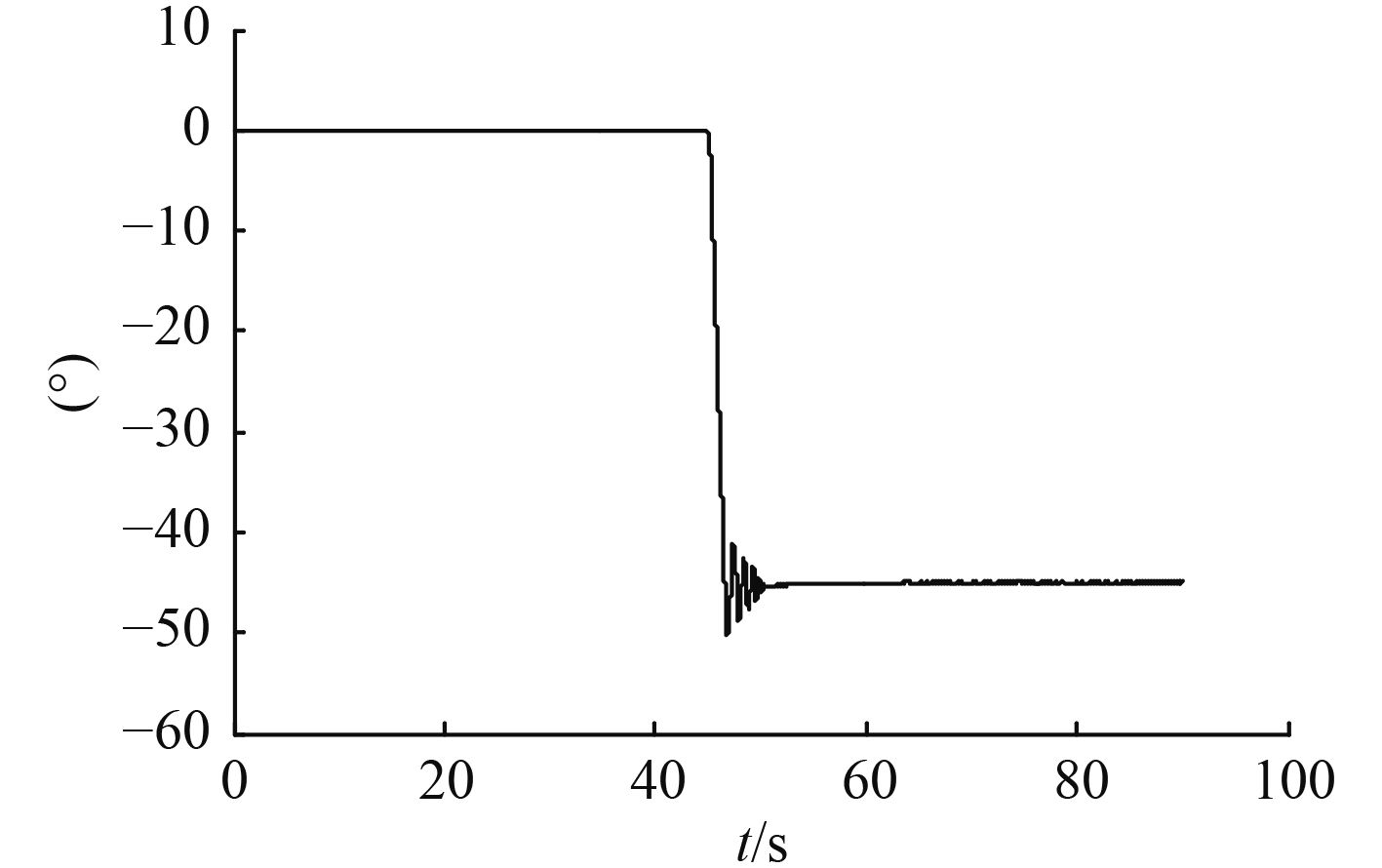图 8 下潜时变化 Fig. 8 Changes when dive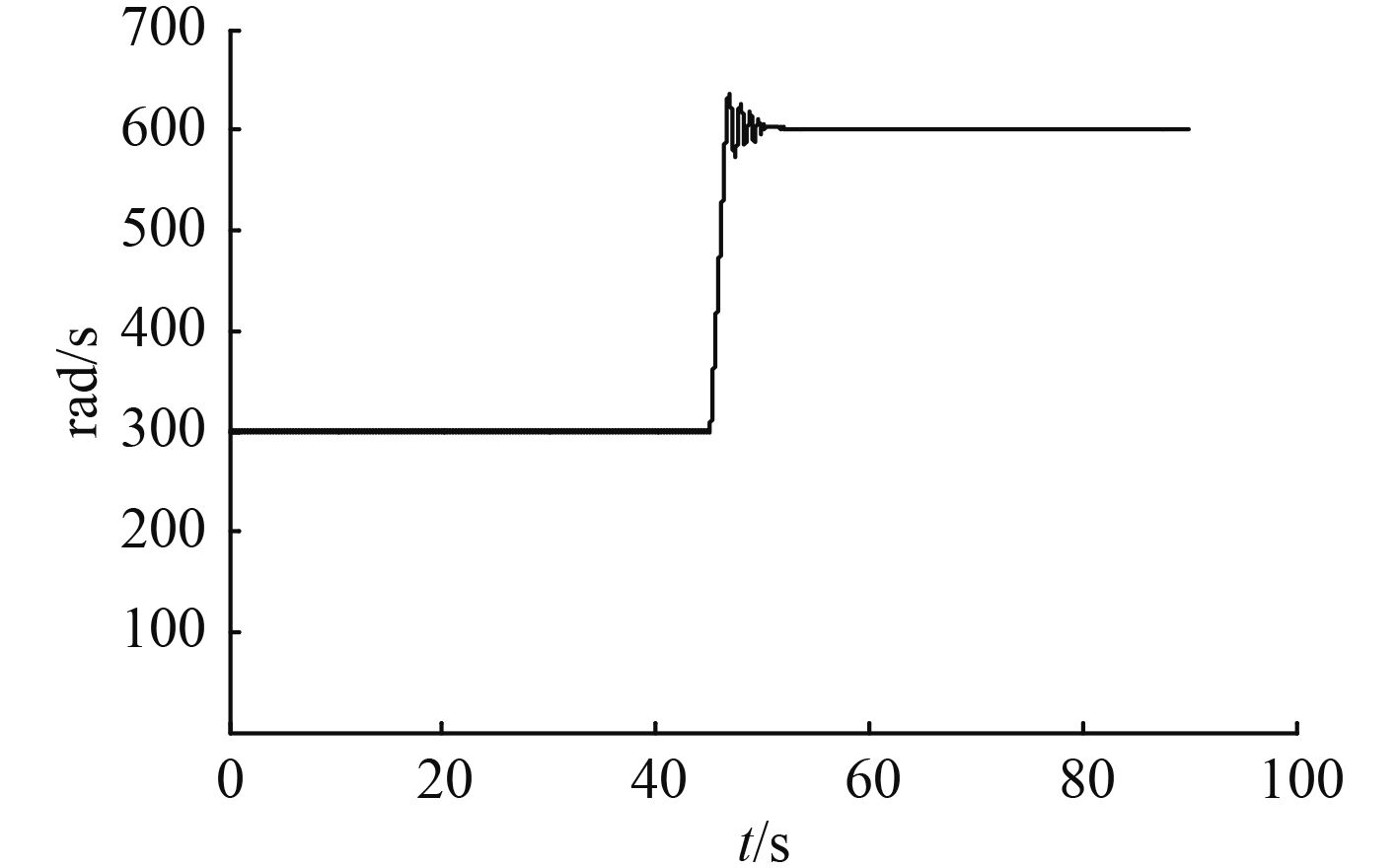图 9 2，3号电机转速变化 Fig. 9 No.2.3 motor speed change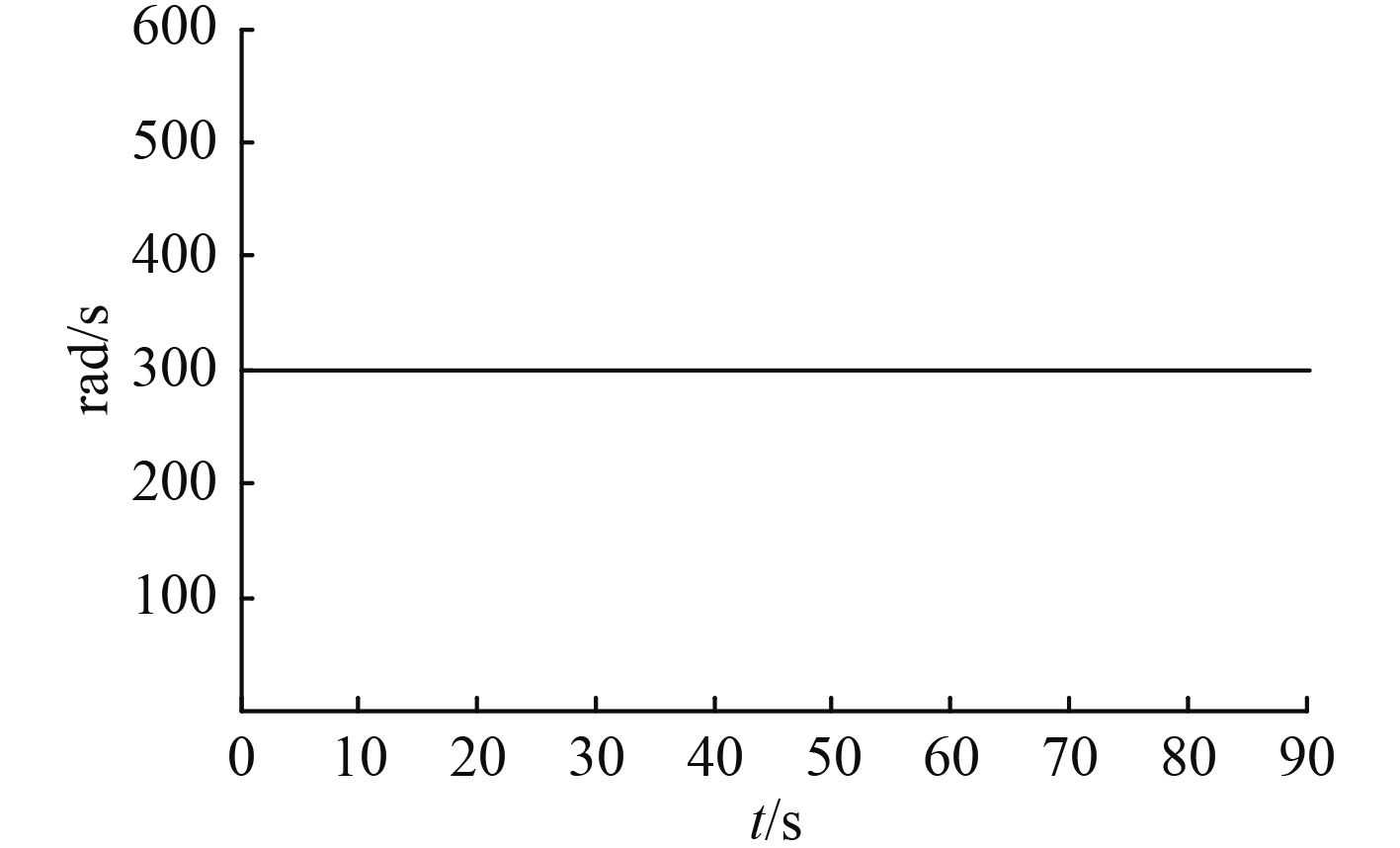图 10 1，4号电机转速变化 Fig. 10 1，4 motor speed changes

4.2 偏转过程仿真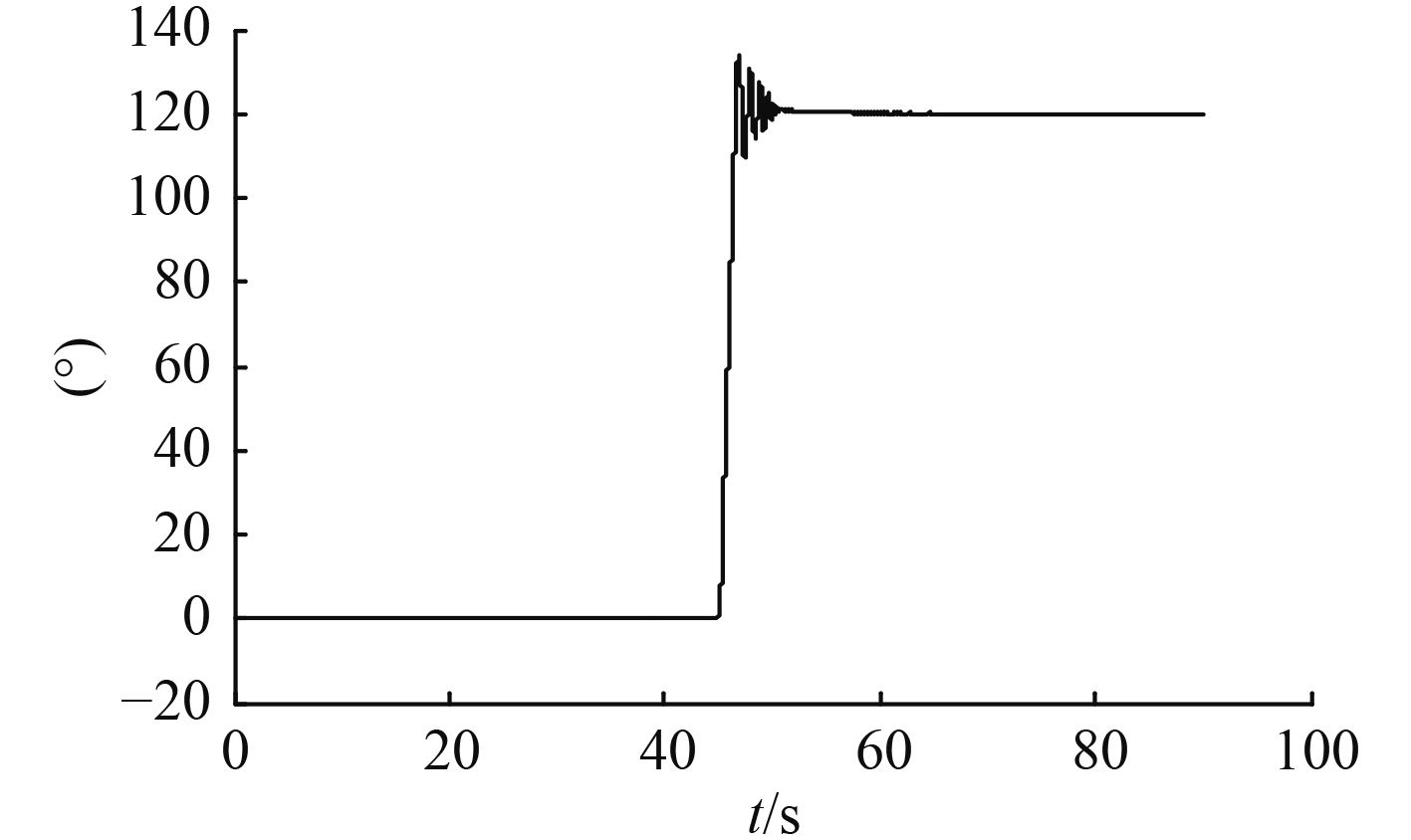图 11 转向时 $\varphi$ 变化 Fig. 11 $\varphi$ change when turning

5 湖　试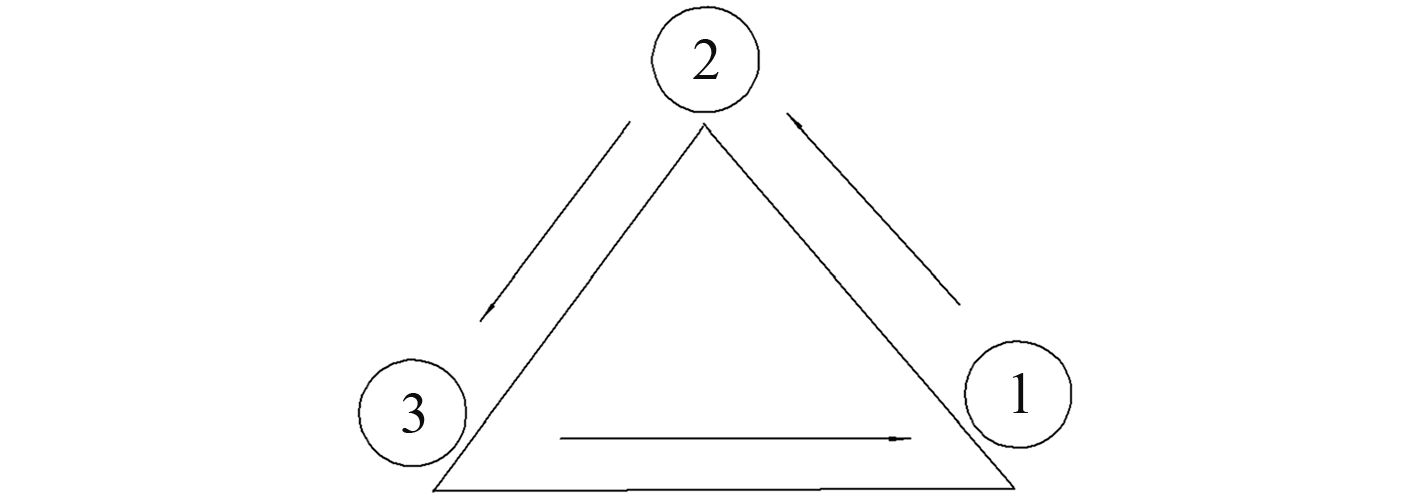图 12 试验路线 Fig. 12 Test route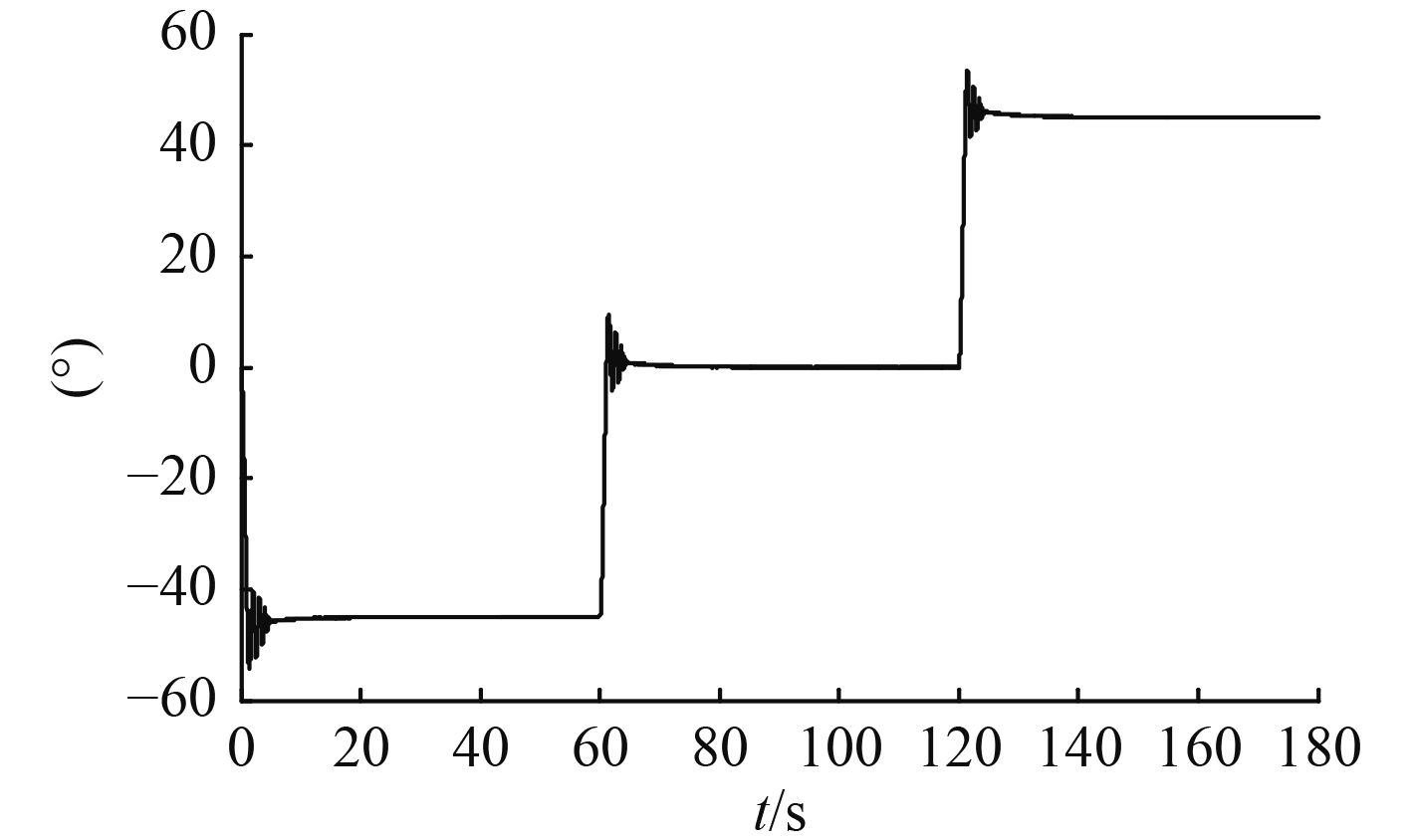图 13 试验过程中θ变化 Fig. 13 θ change during the test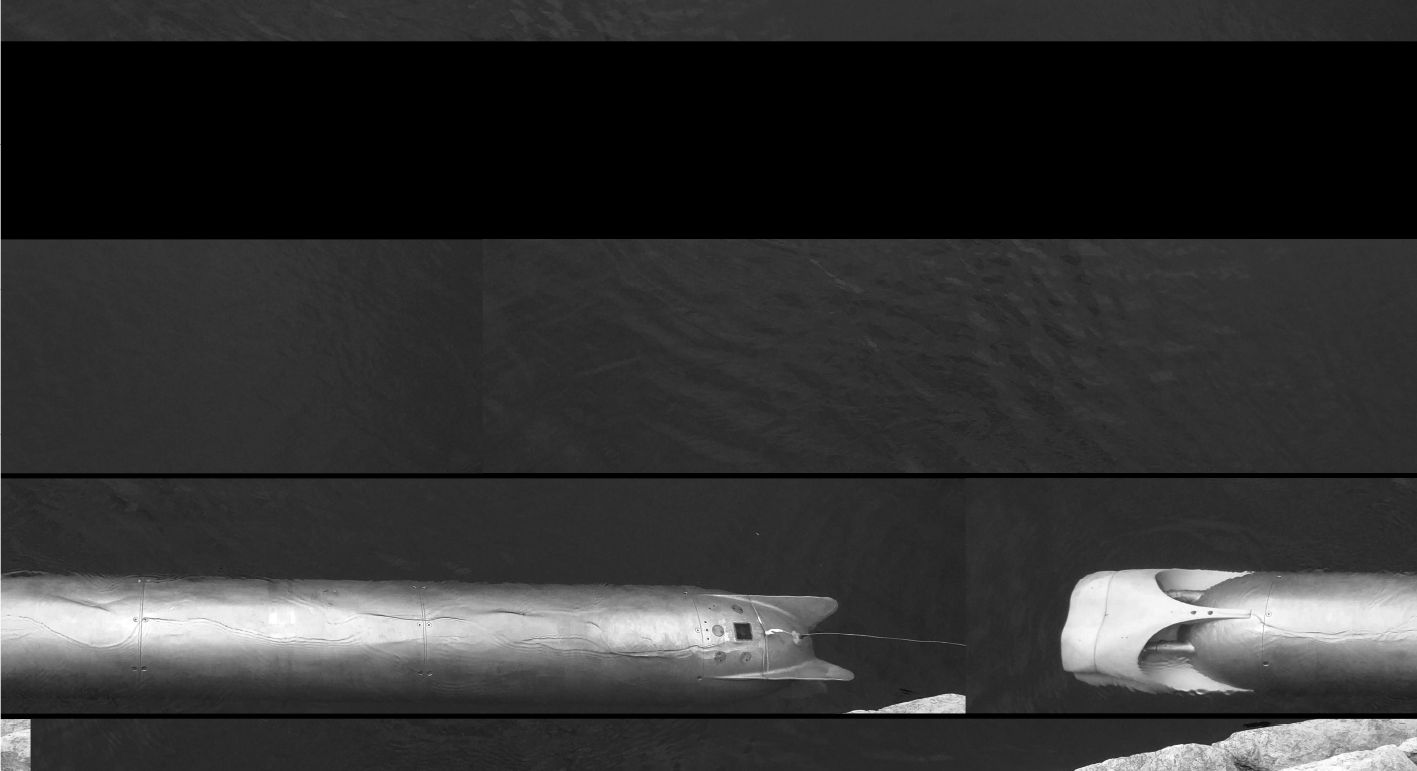图 14 试验现场 Fig. 14 Test site
6 结　语

  WANG S X, SUN X J, WU J G, et al. Motion characteristic analysis of a hybrid-driven underwater glider[C]// OCEANS 2010 IEEE-Sydney. IEEE, 2010: 1–9.  王树新, 刘方, 邵帅, 等. 混合驱动水下滑翔机动力学建模与海试研究[J]. 机械工程学报, 2014, 50 (2): 16–17.  杨庆华, 宋召青. 四旋翼飞行器的建模控制与仿真[J]. 海军工程学院学报, 2009, 24 (5): 485–502.  王晓鸣. 混合驱动水下自航行器动力学行为与控制策略研究[D]. 天津: 天津大学. 2009: 20–25.  ROBERTS G N, SUTTON R. Further advances in unmanned marine vehicles[M]. Piscataway: IET, 2006: 33–35.  JOSHUA G G. Underwater gliders: dynamics, control and design[D]. New Jersey: Princeton University, 2005: 15–17.  SALIH. L, MODELLING and PID controller design for a quadrotor unmanned air vehicle[J]. IEEE AQTR, 2010, 39 (5): 697–699.  陈宇航. 水下滑翔机建模与控制研究[R]. 西安: 西北工业大学, 2009.  蒋新松, 封锡盛, 王棣棠. 水下机器人[M].沈阳: 辽宁科学技术出版社, 2000: 304–311.  郭威, 崔胜国, 赵洋, 等. 一种遥控潜水器控制系统的研究与应用[J]. 机器人, 2008, 30 (5): 5–6.